# Pratica domande sulla somma degli angoli in un triangolo

• Note di Apprendimento
• Revisione degli argomenti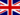Michael W. 2 0 ID 1631014 I can't access this quiz. I don't want to be penalised for not taking the quiz. I need to do all the required tasks in full. Yours Faithfully, Michael.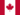Nico G. 2 0 Honestly, what is the point of having quizzes, if you never supply the correct answer? Given that, what is the point of this course, if we never know if we understand it?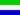Alhassan Paul K. 2 0 I am unable to Practice questions on sum of angles in a triangle. Is there any advice?Jean M B. 1 0 I can open the quizes no problem but how are they corrected. I don't know if i answered right or wrong. So why even bother to add these to the courses if we can't tell how we did?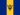Alisa G. 2 0 This becoming really frustrating!!Please fix the problem,we the students are suffering because the quiz aren't opening,please do help us.Alisa G. 1 0 I really hope we the students who CANNOT access the quiz wouldn't be marked as fail,because i would be very upset,help!!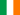Mansoor M. 1 0 The link for practice or quiz is not working, text box appears saying the activity is not supported by Netscape???Rebecca C. 0 0 module: I used the sum of angles in a triangle and isosceles triangle characteristics topic: 60+70-180=50 then 65+65-180=50 (base angles of isosceles triangle are equal )given two sides that are equal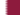Reem Mohammed A. 0 0 how can I check my answer after I finished the quiz?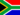Nkululeko M. 0 0 module: I used the sum of angles in a triangle and isosceles triangle characteristics topic: 60+70-180=50 then 65+65-180=50 (base angles of isosceles triangle are equal )given two sides that are equal
• Text Version